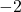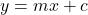Question

Which equation represents the line through (4, -6) and (0, 2)?
y= -2x + 2
y= 2x – 2
y= 2x + 2
y= -2x – 2

1.JezebelThe Correct equation is :
•The given points are :
• (4 , -6)
• (0 , 2)
we can see that the y – intercept (c) is 2, from point (0 , 2)
now, let’s find the slope (m) :
••••now, the equation of line will be :
••2.